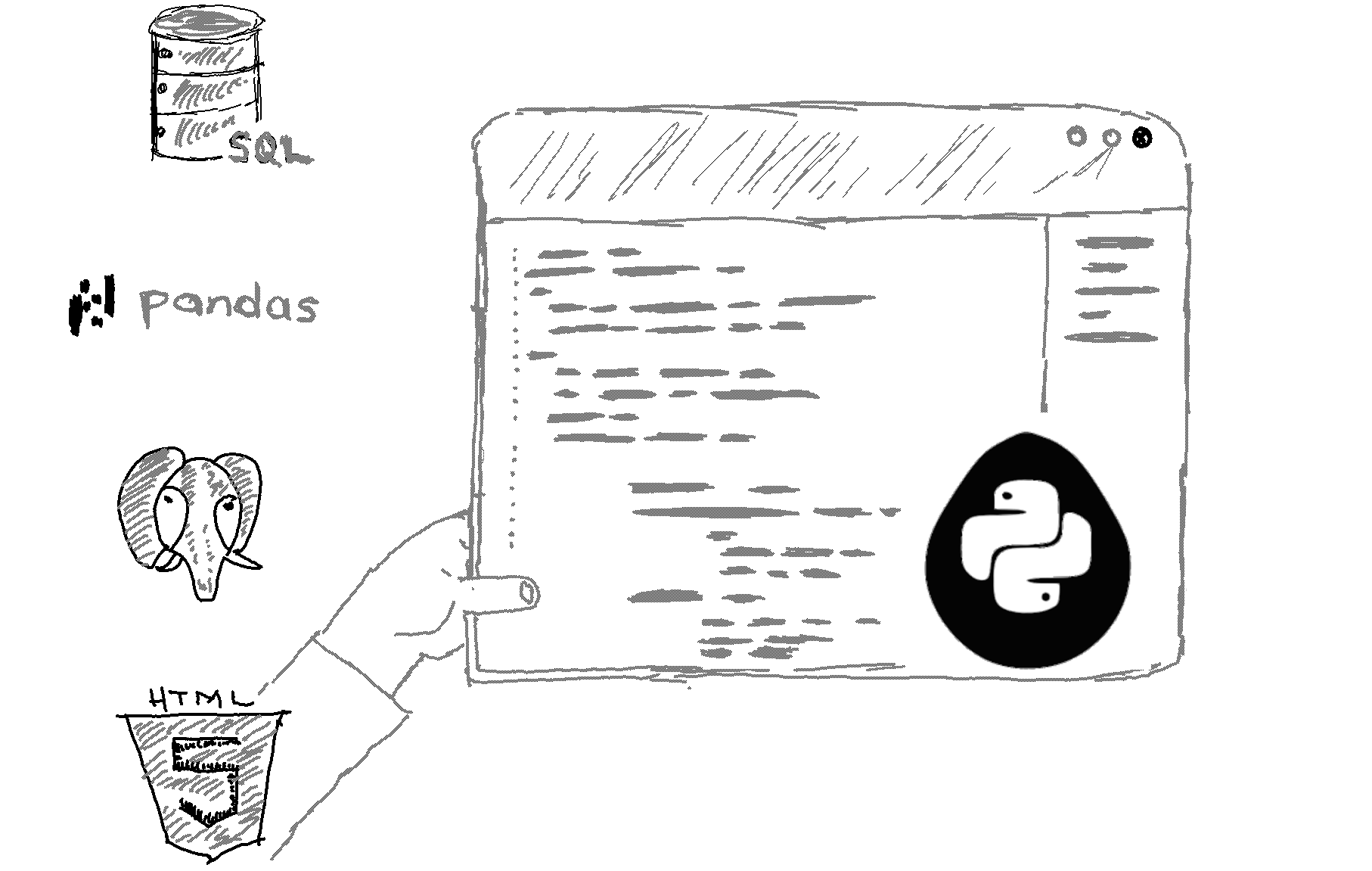# What can I use as a replacement for the switch statement in Python?

In Python, there are several ways to achieve the functionality of a switch statement, which is not a built-in feature of the language. Some common alternatives include:

1. Using if-elif-else statements: This is the most basic and widely used method for implementing a switch-like functionality. Each case is implemented as an elif block, with a final else block to handle any remaining cases:

```def my_function(x):
if x == "case1":
#do something
elif x == "case2":
#do something
else:
#do something
```
2. Using a dictionary of functions: This method uses a dictionary where the keys are the cases and the values are the corresponding functions to be executed:
```def case1():
#do something

def case2():
#do something

def default():
#do something

cases = {
"case1": case1,
"case2": case2
}

def my_function(x):
func = cases.get(x, default)
func()```
3. Using a dictionary of lambda functions: This is similar to the above method, but uses lambda functions instead of named functions:

```cases = {
"case1": lambda: #do something,
"case2": lambda: #do something
}

def my_function(x):
func = cases.get(x, lambda: #do something)
func()
```
4. Using a list of tuples: This method uses a list of tuples, where each tuple contains a case and a corresponding function:
```cases = [
("case1", case1),
("case2", case2)
]

def my_function(x):
for case, func in cases:
if x == case:
func()
break
else:
#do something
```
5. Using the built-in dispatch function of the functools module:
```from functools import dispatch

@dispatch(int)
def my_function(x):
#do something

@dispatch(str)
def my_function(x):
#do something
```
##### Recommended Course

Learn Flask development and learn to build cool apps with our premium Python course on Udemy.